# Diagram of functional elements

circuit of functional elements, network of functional elements

A mathematical model of real objects, related to information processing, in which intermediate results may be used several times. These objects include, for example, electron-tube schemes, neural networks and certain forms of computing algorithms. This is one of the basic classes of control systems (cf. Control system). A diagram of functional elements can be considered as an automaton without memory.

Mathematically, a diagram of functional elements can be defined as an oriented graph without cycles with its edges and vertices marked; its set of vertices can be divided into two subsets. The vertices of one of these are called the inputs of the diagram. No edges can lead to these vertices, and each of them is ascribed a letter of the alphabet of variables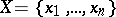. The vertices of the other subset are ascribed letters of the alphabet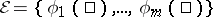of functional symbols.

The alphabet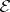is in a one-to-one correspondence with a set of functions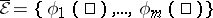. Certain vertices of the graph are distinguished and are called the outputs of the diagram. A vertex at which (numbered) edges come together and which is ascribed the symbolfrom(its valency is equal to the number of edges coming together) is called the functional element. The other ends of the edges that are incident to this vertex are the inputs of, while the vertex itself is the output of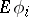. For every given assignment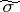at the inputs of, the value ofonis realized at the output of(i.e. at the vertex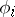); thus, the functional elementrealizes the function. Hence every diagram of functional elements realizes certain functions at their outputs. The set of functional elements that corresponds to the alphabet, from which diagrams of functional elements are constructed, is called the basis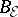. The set of all diagrams of functional elements, constructed using functional elements from, is called the set of diagrams of functional elements in the basis. If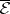is complete, thenis complete, and a diagram of functional elements incan realise any function. It is further assumed that the variables fromtake the values, and thatis a subset of the functions of the algebra of logic. The results for this type of bases are most complete.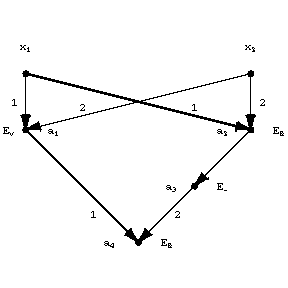Figure: d031570a

The diagram in the basis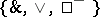(see Fig.) is an example of a diagram of functional elements. Its inputs are the vertices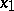and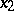, its output the vertex, and at it the functionis realized.

An equivalent definition of a diagram of functional elements can also be given in terms of equalities. For the example in the figure, such a system can be written down in the following way: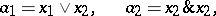Functional elements inare usually ascribed non-negative numbers, called the weights (or complexities) of the functional elements of the basis. By the complexity of a diagram of functional elements one understands the sum of the weights of all functional elements that are present in this diagram. The minimum complexity that is sufficient for the realization of every Boolean function invariables by a diagram of functional elements in any finite basis (with non-zero weights) is asymptotically equal to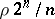(see ), whereis a constant independent of the basis (see Synthesis problems). The minimum complexity that is sufficient for the realization by a diagram of functional elements of a systemof functions dependent on the same variables is asymptotically equal to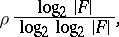where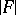is the number of functions inandis a constant calculated for the basis (see ).

According to the number of inputs that can be associated with an output of an arbitrary functional element one can single out so-called diagrams of functional elements without output branching, or formulas, from the class of diagrams of functional elements (the output of each functional element of such a diagram can only have one input associated with it). Unlike formulas, diagrams of functional elements of general form can be considered as calculation diagrams which take intermediate results into account. For formulas, the minimum complexity necessary for the realization of every Boolean function invariables by a formula in any finite basis (with non-zero weights) is asymptotically equal to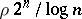, whereis a constant depending on the basis (for a comparison with contact-schemes, see Contact scheme). For bases that contain elements with zero weights, the complexities of diagrams of functional elements behave differently (see, for example, ).

Furthermore, the problem of synthesis of diagrams in infinite bases is of interest. The most completely studied case is the one in which the elements of the basis realise threshold functions. A Boolean function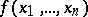is a threshold function if there are real numbersandsuch that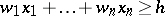(*)

if and only if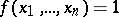. A functional element that realizes a threshold function is called a threshold element. Diagrams of functional elements in a basis of threshold elements are called diagrams of threshold elements. Two types of such bases are usually studied: 1) the weights of the threshold elements are equal to 1; or 2) the weight of a threshold element is equal to the sum of the absolute values of all coefficients(given the condition that the threshold functions are defined by the integer inequality (*)). For all these bases asymptotic estimates have been obtained for the complexity of diagrams of threshold elements: 1)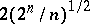(see ); and 2)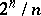(see ).

The path between the input and output of a diagram of functional elements is called a chain. The number of vertices of the chain, apart from the input, is called the length of the chain. The maximum length of a chain in a diagram of functional elements is called the depth of the diagram. The minimum depth of a diagram of functional elements (or of a formula) that is sufficient for the realization of every Boolean function invariables in the basisis equal to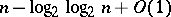(see ).

Apart from weights, the functional elements of the basis can be ascribed non-negative numbers, called delays. By the delay of a chain one means the sum of the delays of the functional elements in it. By the delay of a diagram of functional elements one means the maximum delay of the chains of this diagram. The concepts of a delay (for unit delays of the basis) and the depth of diagrams of functional elements, generally speaking, do not coincide (see ).

The power of a diagram can also be taken as the complexity of the diagram. The power of a diagram of functional elements on an assignmentof input variables is the number of its functional elements the outputs of which are equal to 1. The power of a diagram of functional elementsis the maximum of its powers onfor all possible. The minimal power that is sufficient for the realization of every Boolean function invariables by a diagram of functional elements in an arbitrary finite basis is of an order no less thanand no more than(see , ).